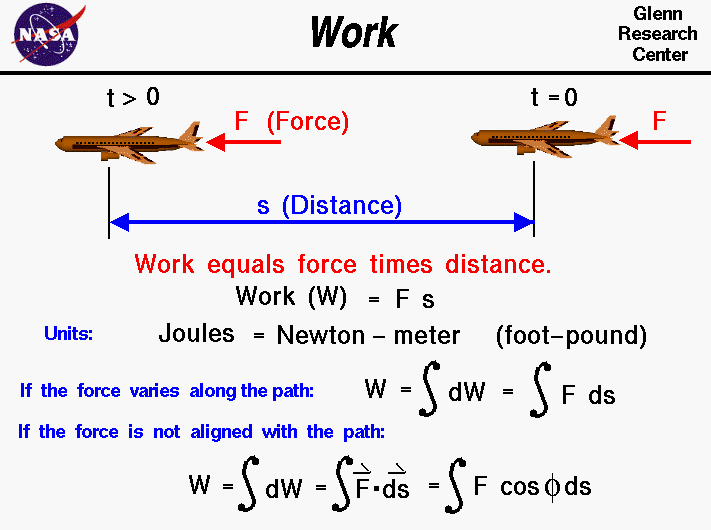+ Text Only Site
+ Non-Flash Version
+ Contact GlennThermodynamics is a branch of physics which deals with the energy and work of a system. Thermodynamics deals only with the large scale response of a system which we can observe and measure in experiments. As aerodynamicists, we are most interested in the thermodynamics of propulsion systems and high speed flows. The first law of thermodynamics deals with the relationship between heat, energy, and work. We all have a basic understanding of work in general, but scientists have a more precise definition of work. For scientists, work is the product of a force acting through a distance. As an example shown on the slide, an aircraft which is acted on by a force F from time equals zero to some later time t moves some distance s. The work W done on the aircraft during this time is: W = F * s The units of work is the Joule, which is equal to a Newton - meter. In the English system the unit of work is the foot - pound. In our simple example, the force is a constant value aligned with the displacement of the aircraft. In most real applications, the force varies along the flight path. Work is then the integrated value of the force along the path. If we use the symbol S [ ] ds for the integral: W = S [F] d s The force is a vector quantity which changes in both magnitude and direction. The work is then equal to the integrated value of the component of the force in the direction of the displacement. If the angle between the force and displacement equals phi, then the work is given by: W = S [F * cos(phi)] d s where cos is the trigonometric cosine function. It is important to note that work is only done on, or by the component of the force along the path. Using a cruising aircraft as another example, the lift is defined to be the force perpendicular to the flight path. Lift does no work on a cruising aircraft because the displacement is perpendicular to the force. Similarly, if an aircraft was stopped at the end of the runway with the brakes on and the engines at full throttle, the engines do no work on the aircraft because the displacement distance is zero. In the previous examples, we have been concerned with the work done on, or by a force applied to a solid object. For a gas, work is done when the volume of a gas is changed at some pressure since pressure represents a force per unit area and volume can be related to the surface area and a distance. Notice that to produce work, the volume must change. Activities: Guided ToursThermodynamics:Navigation ..Beginner's Guide Home Page+ Inspector General Hotline + Equal Employment Opportunity Data Posted Pursuant to the No Fear Act + Budgets, Strategic Plans and Accountability Reports + Freedom of Information Act + The President's Management Agenda + NASA Privacy Statement, Disclaimer, and Accessibility CertificationEditor: Nancy Hall NASA Official: Nancy Hall Last Updated: May 05 2015 + Contact Glenn